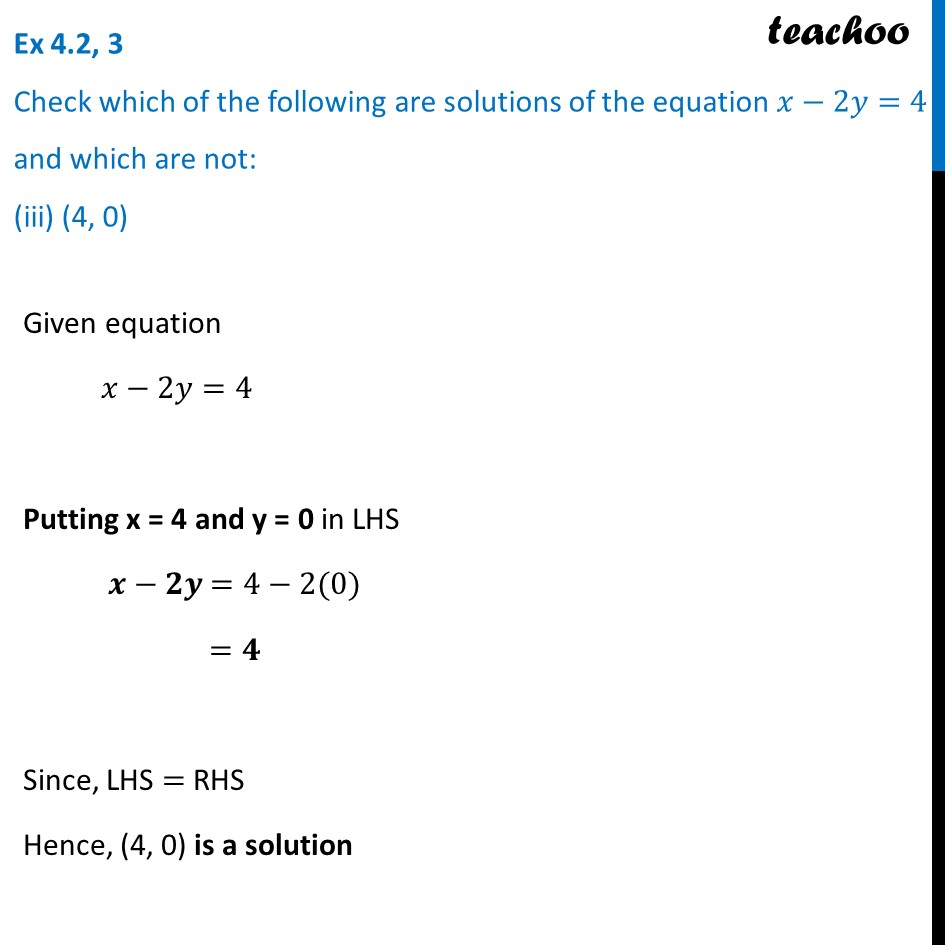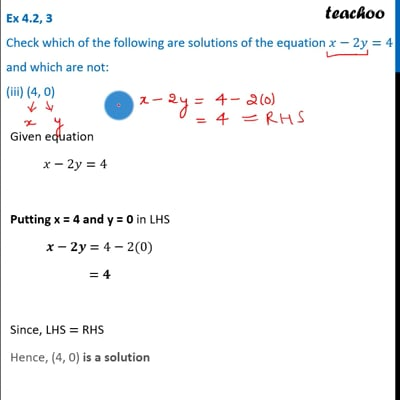Ex 4.2

Chapter 4 Class 9 Linear Equations in Two Variables
Serial order wiseThis video is only available for Teachoo black users

Introducing your new favourite teacher - Teachoo Black, at only ₹83 per month

### Transcript

Ex 4.2, 3 Check which of the following are solutions of the equation 𝑥−2𝑦=4 and which are not: (iii) (4, 0) Given equation 𝑥−2𝑦=4 Putting x = 4 and y = 0 in LHS 𝒙−𝟐𝒚 =4−2(0) =𝟒 Since, LHS = RHS Hence, (4, 0) is a solution